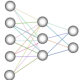# Javascript Multi-Dimentional K-Means Algorithm

June 10, 2010

Having created a Javascript k-means function to process a single dimentional array I moved on to working out how to process a multi-dimentional array. The basic premise is the same, but you have to loop through the additional arrays and use a more advanced Euclidean n-space formula to calculate which cluster to allocate the point to.

Below is my k-means clustering learning machine algorithm.

```function kmeans( arrayToProcess, centroids, clusters )
{

Groups=Array();
iterations=0;

function getType(o)
{
var _t;
return ((_t = typeof(o)) == "object" ? o==null && "null" || Object.prototype.toString.call(o).slice(8,-1):_t).toLowerCase();
}

function deepCopy(target,source)
{
for(var p in source)
{
if(getType(source[p])=="array"||getType(source[p])=="object")
{
target[p]=getType(source[p])=="array"?[]:{};
arguments.callee(target[p],source[p]);
}
else
{
target[p]=source[p];
}
}
}

tempdistance=0;
oldcentroids=[];
deepCopy( oldcentroids, centroids );

do
{

for( reset=0; reset < clusters; reset++ )
{

Groups[reset]=Array();

}

changed=false;

for( i=0; i < arrayToProcess.length; i++)
{

lowdistance=-1;
lowclusters=0;

for( clustersloop=0; clustersloop < clusters; clustersloop++ )
{

dist=0;

for( j=0;  j < arrayToProcess[i].length; j++ )
{

dist+=Math.pow( Math.abs( arrayToProcess[i][j] - centroids[clustersloop][j] ), 2 );

}

tempdistance=Math.sqrt( dist );

if ( lowdistance==-1 )
{

lowdistance=tempdistance;
lowclusters=clustersloop;

}
else if ( tempdistance <= lowdistance )
{

lowclusters=clustersloop;
lowdistance=tempdistance;

}

}

Groups[lowclusters].push( arrayToProcess[i].slice() );

}

for( clustersloop=0; clustersloop < clusters; clustersloop++)
{

for( i=0, totalGroups=Groups[clustersloop].length; i < totalGroups; i++ )
{

for( j=0, totalGroupsSize=Groups[clustersloop][i].length; j < totalGroupsSize; j++ )
{

centroids[clustersloop][j]+=Groups[clustersloop][i][j]

}

}

for( i=0; i < centroids[clustersloop].length; i++ )
{

centroids[clustersloop][i]=( centroids[clustersloop][i]/Groups[clustersloop].length );

if ( centroids[clustersloop][i]!=oldcentroids[clustersloop][i] )
{

changed=true;
oldcentroids=[];
deepCopy( oldcentroids, centroids );

}

}

}

iterations++;

}
while(changed==true);

return Groups;

}```

Filed under: Mathematics,Programming — Tags: , , , , , , — admin @ 9:32 pm

1.Most cool, my friend!

Comment by Jim — December 24, 2011 @ 2:37 pm

2.can you post a complete example to show us how it works, or comment about what the input data you expected

Comment by horsley — June 4, 2013 @ 7:43 am

3.Hiya,

I’ve just been looking at the code and I was going to upload a method however I’ve found a bug and I want to fix it first.

Comment by admin — June 5, 2013 @ 9:32 pm

4.Yeah, I found a bug too.
I found that the amount of iterations always was one.

On the line 05, you want to save centroids to oldcentroids
but you simply use the assign statement.
In javascript, array won’t be copied by assign statement,
but they share the same address.

It’s means that when you calc the new centroids, you also overwrite the old_centroids
(You can trace that bro), so when you determine if centroids changed, it always be true.

The solution is copy the old centroids with other methods like Array.slice()

Sorry for my poor English

Comment by horsley — June 18, 2013 @ 4:14 am

5.Both slice, concat were not working to copy the value correctly.
Because that the multi dimention array in javascript is a one dimention array which items was a reference to the second dimention.
I found a solution.

function getType(o)
{
var _t;
return ((_t = typeof(o)) == “object” ? o==null && “null” || Object.prototype.toString.call(o).slice(8,-1):_t).toLowerCase();
}
function deepCopy(target,source)
{
for(var p in source)
{
if(getType(source[p])==”array”||getType(source[p])==”object”)
{
target[p]=getType(source[p])==”array”?[]:{};
arguments.callee(target[p],source[p]);
}
else
{
target[p]=source[p];
}
}
}

use deepCopy to save old_centroids

Comment by horsley — June 18, 2013 @ 5:34 am

6.I also found some problem with the distance calculate on line 33 and below
you are doing sqrt(abs(a^2 – b^2))
It should be sqrt((abs(a – b))^2)

Comment by horsley — June 18, 2013 @ 6:06 am

7.Is there a fully debugged version?

Comment by Jm — July 27, 2013 @ 1:45 am

8.Hello,

Alas I’ve been a little busy just recently and haven’t had a chance to fix it. Hopefully I’ll have to sometime tomorrow.

Comment by admin — July 28, 2013 @ 11:58 am